## Friday, October 6, 2017

### New old package for population genetic simulation and numerical simulation, PopGen

I just posted a simple R package called PopGen to my GitHub. The package does population-genetic & game theory simulations & numerical analyses in R. I wrote most of the functions of this package way back in 2012 and it has been available since then from my personal website (under the name popgen which I have changed to PopGen because the former matches an existing, but no longer maintained, CRAN R package).

Many, but not all, of the functions of the package are based on models from the book Evolutionary Theory: Mathematical and Conceptual Foundations published in 2004 by Sean Rice.

The package can already be installed using devtools as follows:

``````library(devtools)
install_github("liamrevell/PopGen")
``````
``````library(PopGen)
``````

Here are a few of the functions in the package:

`founder.event`: This function simulates genetic drift with a founder event at a particular time. The population rapidly expands after the founder event. The user can visualize either the frequency of the 'A' allele or the genetic variance through time. Note that the variance will generally decrease during a founder event, but sometimes it can increase, for instance if the rarer allele happens to increase in frequency in the population:

``````set.seed(4)
founder.event(p0=0.1,Ne=1000,Nf=2)
``````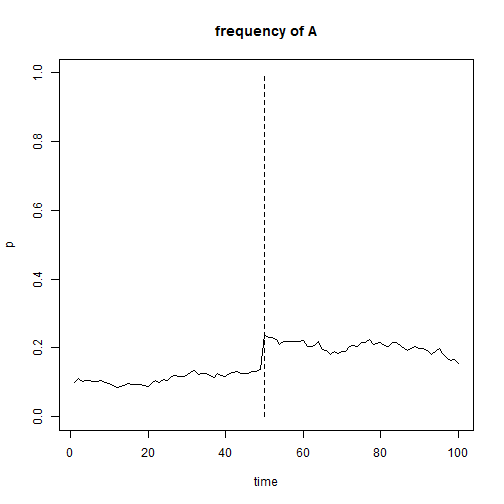``````set.seed(4)
founder.event(p0=0.1,Ne=1000,Nf=2,show="var")
``````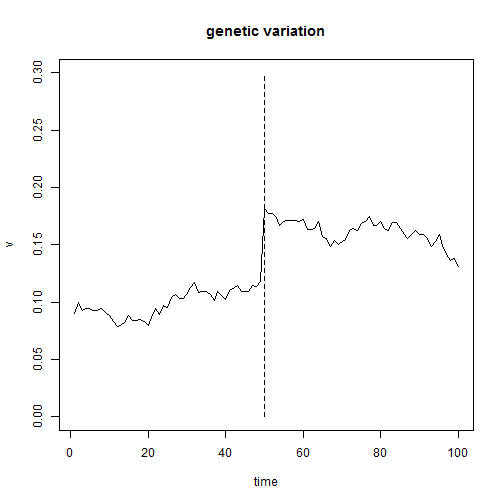`freqdep`: This function does numerical analysis of a frequency dependent selection model in which heterozygotes experience negative selection when they are common. This model is neat because though it is fully deterministic it can result in chaotic behavior for certain values of frequency dependent selection against heterozygotes.

``````freqdep(time=100)
``````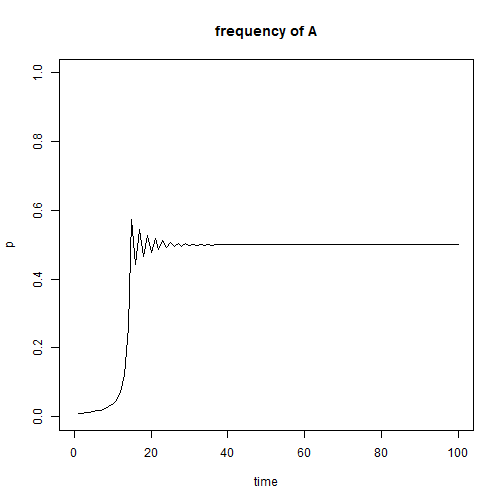``````freqdep(s=1.5,time=100)
``````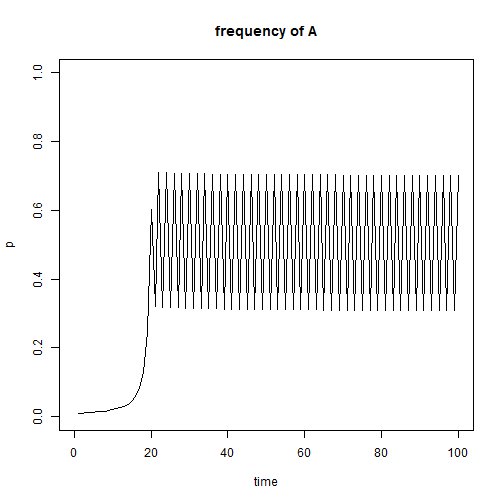``````freqdep(s=2,time=100) ## chaotic
``````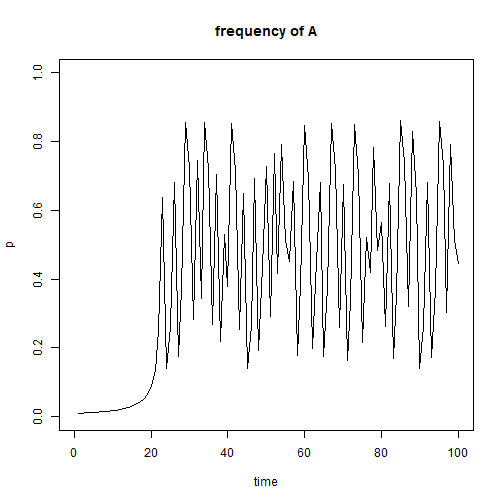We can also visualize this using a cobweb plot as follows:

``````freqdep(s=2,time=500,show="cobweb",pause=0.01) ## chaotic
``````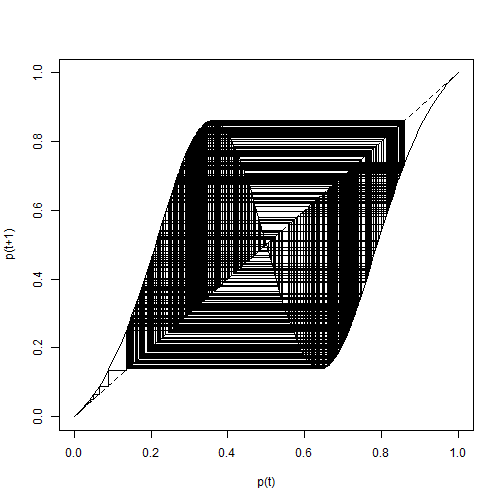`genetic.drift`: This function simulates genetic drift at a biallelic genetic locus with no selection and no mutation in a sexually reproducing diploid population or set of populations. For instance:

``````set.seed(1)
genetic.drift(p0=0.5,Ne=20,nrep=10,time=100,show="p",pause=0.1)
``````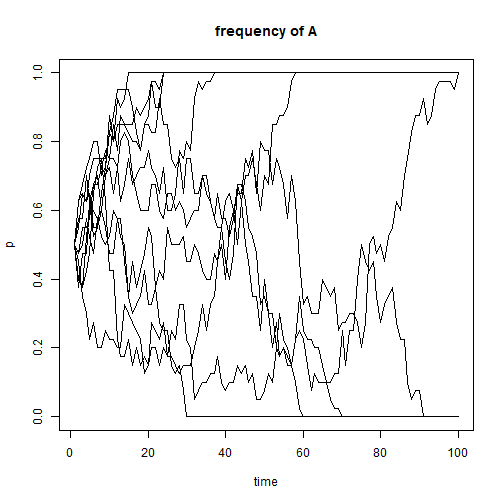We can also show the decline in heterozygosity through time under genetic drift compared to a theoretical expectation:

``````set.seed(1)
genetic.drift(p0=0.5,Ne=20,nrep=10,time=100,show="heterozygosity",pause=0.1)
``````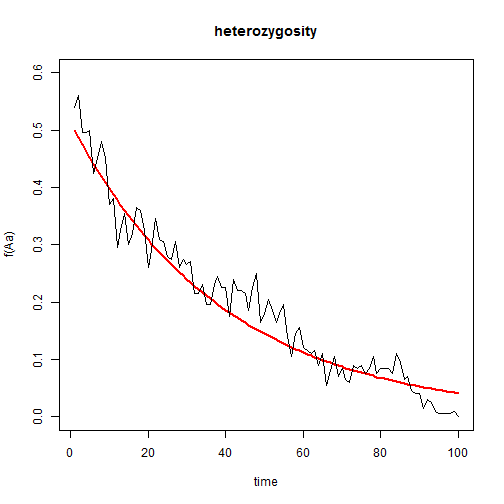or show a histogram of the relative frequencies of each genotype through time:

``````genetic.drift(p0=0.5,Ne=20,nrep=10,time=100,show="genotypes",pause=0.1)
``````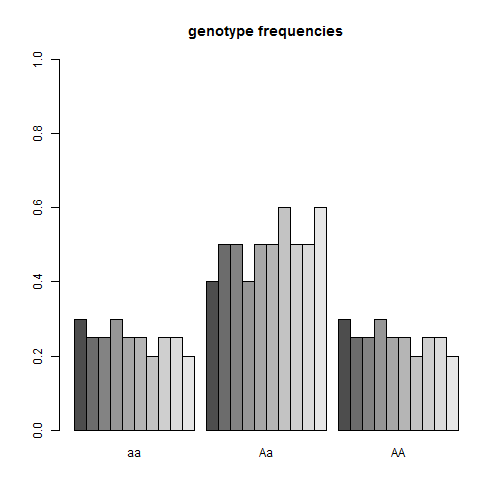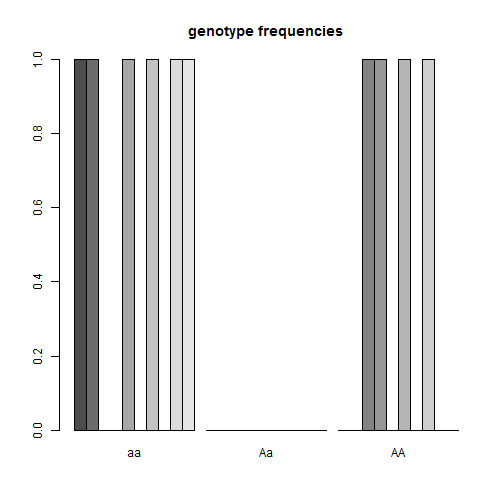(This is the coolest, but needs to be animated to be appreciated.)

`hawk.dove`: This function performs numerical analysis of a discrete-time hawk-dove model in which “payoff” determines relative fitness in the population. E.g.:

``````hawk.dove(time=100) ## hawk goes to fixation
``````
``````## Pay-off matrix:
``````
``````##      hawk dove
## hawk  0.6  1.5
## dove  0.5  1.0
``````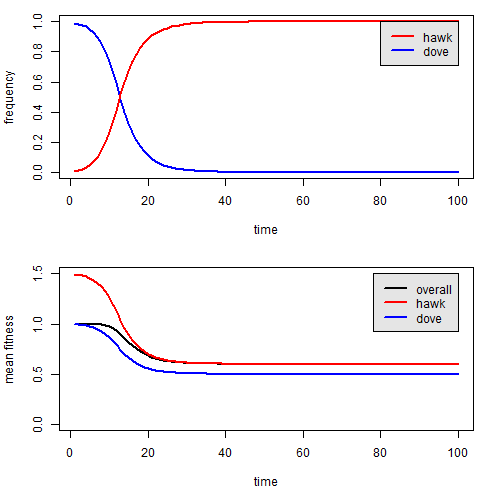``````M<-matrix(c(0.5,0.6,1.5,1),2,2)
hawk.dove(time=100,M=M) ## stable coexistence of hawk & dove
``````
``````## Pay-off matrix:
``````
``````##      hawk dove
## hawk  0.5  1.5
## dove  0.6  1.0
``````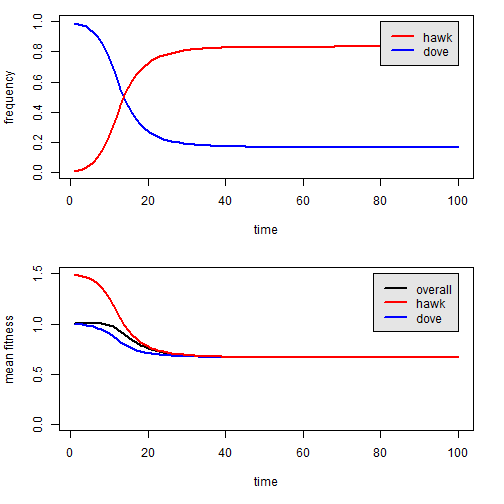`selection`: This function performs numerical analysis of a simple biallelic selection model. We can visualize gene frequencies through time, a cobweb plot, or the fitness surface. For instance:

``````selection(w=c(1.0,0.8,0.8),time=500) ## dominance selection
``````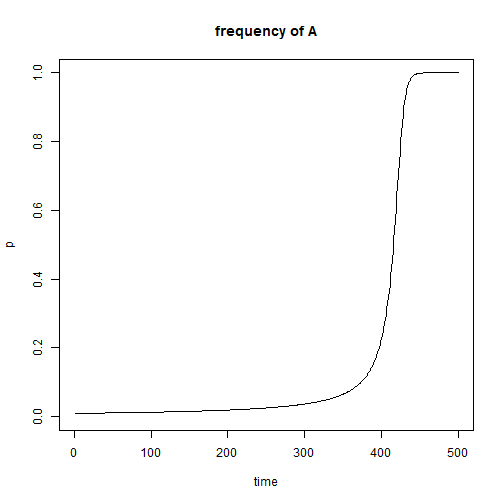``````selection(w=c(0.9,1.4,0.7),time=500) ## heterozygous advantage
``````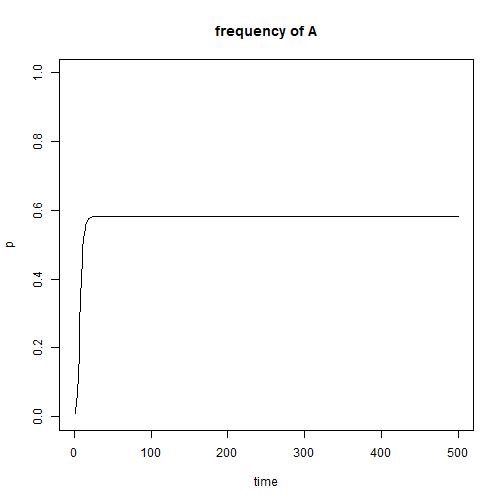``````selection(w=c(0.9,1.4,0.7),time=500,show="cobweb")
``````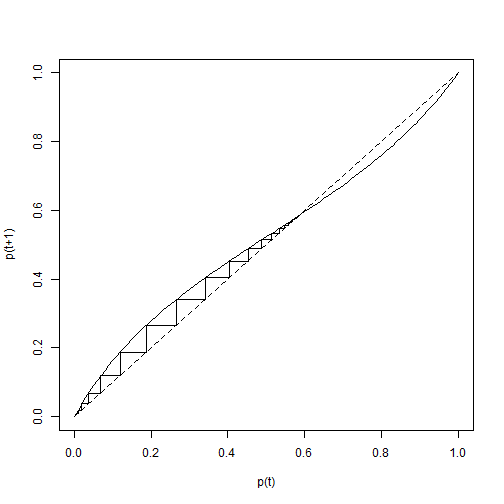The package also includes a few other functions, including one that I developed for a (theoretically in revision) paper with Manuel Leal a few years ago to examine reproductive character displacement in an ecological community.

That's all.